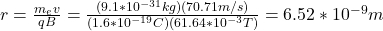## An electron follows a helical path in a uniform magnetic field given by:B =(20i^−50j^−30k^)mTAt time t = 0, the electron’s velocity is given

Question

An electron follows a helical path in a uniform magnetic field given by:B =(20i^−50j^−30k^)mTAt time t = 0, the electron’s velocity is given by:⃗v=(40i^−30j^+50k^)m/sa. What is the angle ϕ between v and B. The electron’s velocity changes with time. Do b. its speed c. the angled. What is the radius of the helical path?

in progress 0
2 months 2021-08-01T04:34:02+00:00 1 Answers 5 views 0

a) 1.38°

b) 7.53*10^11 m/s/s

c) 6.52*10^-9m

Explanation:

a) to find the angle you can use the dot product between two vectors: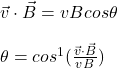v: velocity of the electron

B: magnetic field

By calculating the norm of the vectors and the dot product and by replacing you obtain: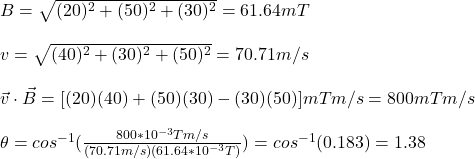the angle between v and B vectors is 1.38°

b) the change in the speed of the electron can be calculated by the change in the momentum in the following way: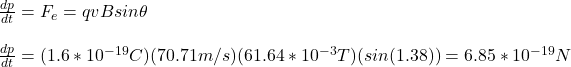due to the mass of the electron is a constant you have: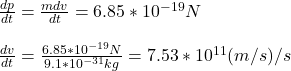the change in the speed is 7.53*10^{11}m/s/s

c) the radius of the helical path is given by: